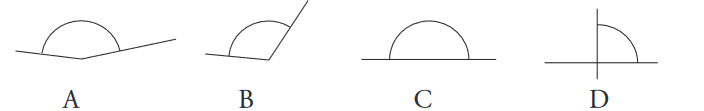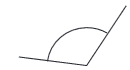# ATSA Angles Practice Test 2023: Air Traffic Controller Aptitude Test

ATSA Angles Practice Test 2023: Air Traffic Controller Aptitude Test: Try our free Air Traffic Selection and Training Aptitude Test (AT-SAT) Angles review question answers. It will help your ATSA Test Prep better. “Air traffic controllers must recognize angles and perform calculations on those angles. The Angles test measures the ability to perform these tasks.” (FAA pamphlet, p. 6) FAA Air Traffic Control System.

ATSA Angles Practice Test in Air Traffic Selection and Training (AT-SAT) Examination is an important part. The Angles test measures the subject’s ability to recognize the measurement of angles. This test contains 30 multiple-choice questions with four response options; the goal is to work quickly and accurately (you’ll have about 10 minutes).

There are two types of questions on the test. The first type presents a picture of an angle and requires the subject to estimate (in degrees) the correct size of the angle (see Figure 3-7). The second type presents a measure in degrees (e.g., 35°) and asks the subject to choose the depicted angle that best represents that degree measurement (Heil and Agnew, 2000, p. 4).

## ATSA Angles Practice Test 2023

 Test ATSA Practice Test 2023 Exam Name Air Traffic Selection and Training Section Angles Question Types Multiple-Choice Test Type Sample Practice Test Total Items 15 Time Limit N/A

Q1.  Which angle is 45 degrees?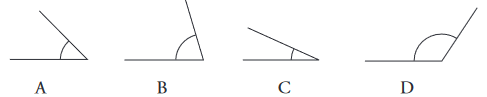Q2. Which angle is 70 degrees?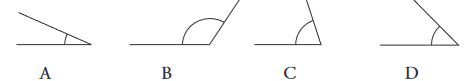Q3. This angle is ____ degrees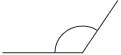• A. 90
• B. 125
• C. 145
• D. 70

Q4. This angle is ____ degrees.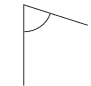• A. 90
• B. 125
• C. 145
• D. 70

Q5. Which angle is 15 degrees?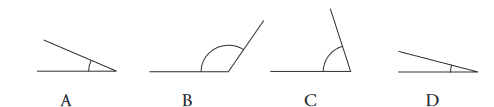Q6. Which angle is 125 degrees?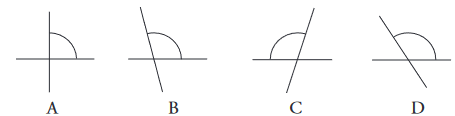Q7. This angle is ____ degrees.• A. 105
• B. 65
• C. 95
• D. 135

Q8. Which angle is 35 degrees?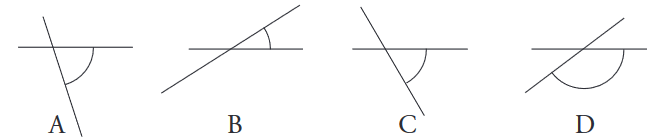Q9. This angle is ____ degrees.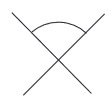• A. 75
• B. 100
• C. 90
• D. 10

Q10. Which angle is 55 degrees?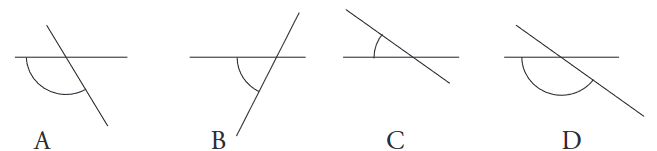Q11. This angle is ____ degrees.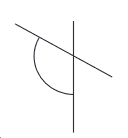• A. 90
• B. 100
• C. 120
• D. 145

Q12. Which angle is 35 degrees?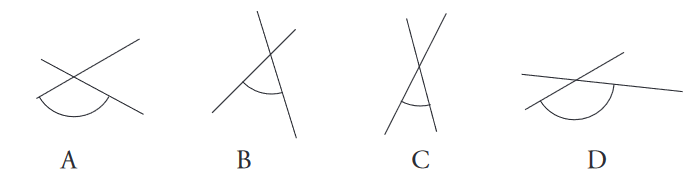Q13. Which angle is 145 degrees?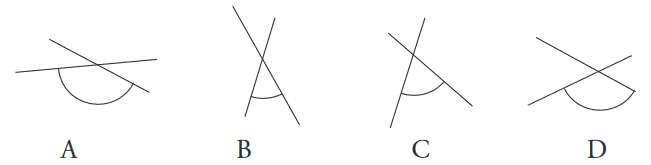Q14. Which angle is 180 degrees?